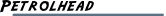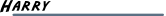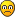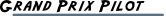[SOLVED] Two final drive ratios

Help on issues you run into with LapTimer; in case you have a question on how to use LapTimer, use the forum "Using LapTimer" instead
snowfree52Posts: 10
Joined: Sun Nov 13, 2011 12:19 am

[SOLVED] Two final drive ratios

One of my cars is a golf and it seems there are two differents drive ratios by gearbox :

one for 1, 2, 3 and 4th gear
a second for 5 et 6th

I wonder how is that possible and if there is a way to implement that in the laptimer ?

Thanks
HarryPosts: 9525
Joined: Sun Sep 12, 2010 10:32 am
Location: Siegum, Germany
Contact:

Re: Two final drive ratios

Hi,

Frequent question. To map this, simply calculate the full ratio (drive + gear) for each gear and enter these value for the gear ratio. In addition, set the drive ratio to 1.

- Harry
snowfree52Posts: 10
Joined: Sun Nov 13, 2011 12:19 am

Re: Two final drive ratios

Thank you very much

Since I found the "how to" by myself :

formula is: (final drive ratio * gear ratio) /2

and put 2 as the final drive (because the app doesn't accept more than 10 as gear ratioedit : I have a problem :

since I have a car with the max power at low RPM, the app gives me max speeds that are way too slow (for 6th speed there is a 45kmh difference)fluppie00Posts: 40
Joined: Sun Jun 26, 2011 9:58 pm
Location: Antwerp, Belgium

Re: Two final drive ratios

I've driven a Audi S3 for a week, this car has also 2 drive ratios, I recalculated the drive ratios like this:

I've multiplied all the gear ratios with the final drive ratio and then the results from 5th and 6th I divided by 4.24.
Like this I calculated the 5th and 6th for a 4.24 final drive ( The 'real' final drive in 5th and 6th is 3.27 ). Should work fine !HarryPosts: 9525
Joined: Sun Sep 12, 2010 10:32 am
Location: Siegum, Germany
Contact:

Re: Two final drive ratios

snowfree52 wrote:edit : I have a problem :

since I have a car with the max power at low RPM, the app gives me max speeds that are way too slow (for 6th speed there is a 45kmh difference)HI again,

max is calculated for the rpm max power is developed. You need to read is exactly like this. With the data given, it is not possible to calculate max speeds for every configuration available in the market. To achieve this, I'd need to add a sketch pad to draw any picture- Harry
snowfree52Posts: 10
Joined: Sun Nov 13, 2011 12:19 am

Re: Two final drive ratios

@fluppie00 : thank you.

@harry : should I put the max power at max RPM then ? does it affect the way the app records laptimes of anything else ?
Harry